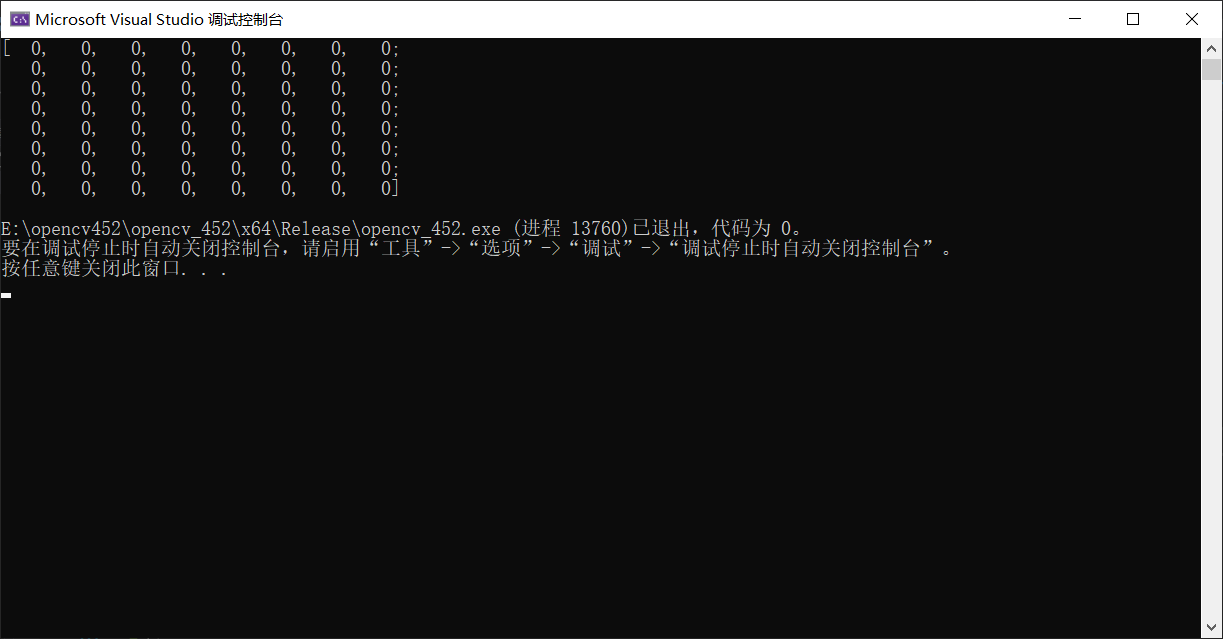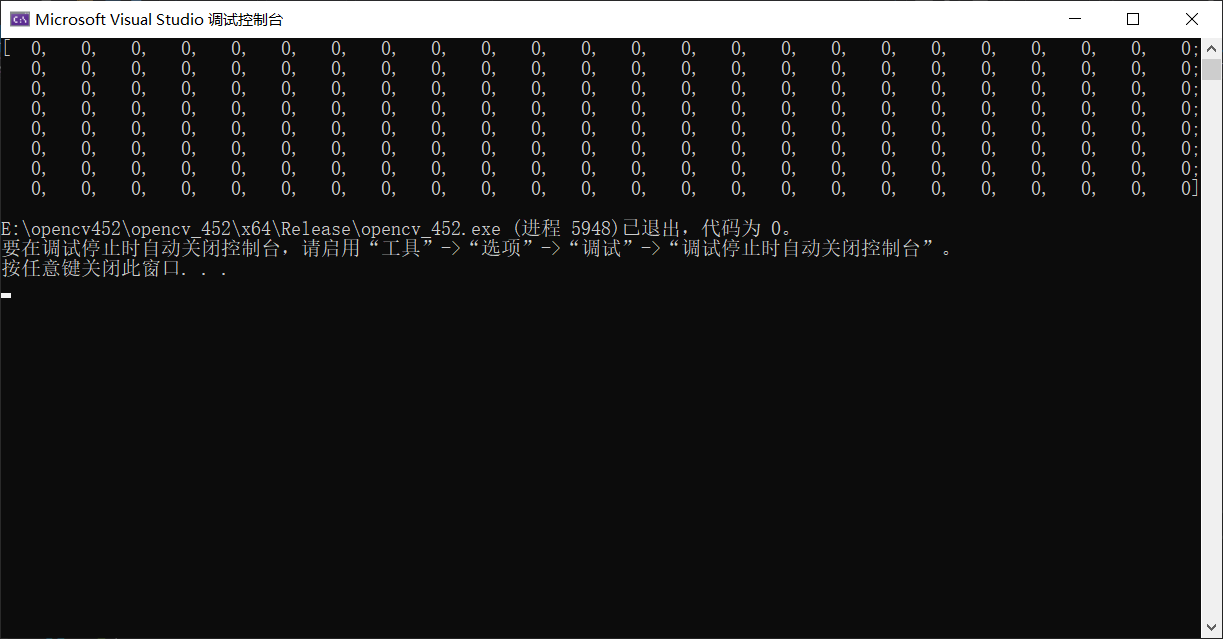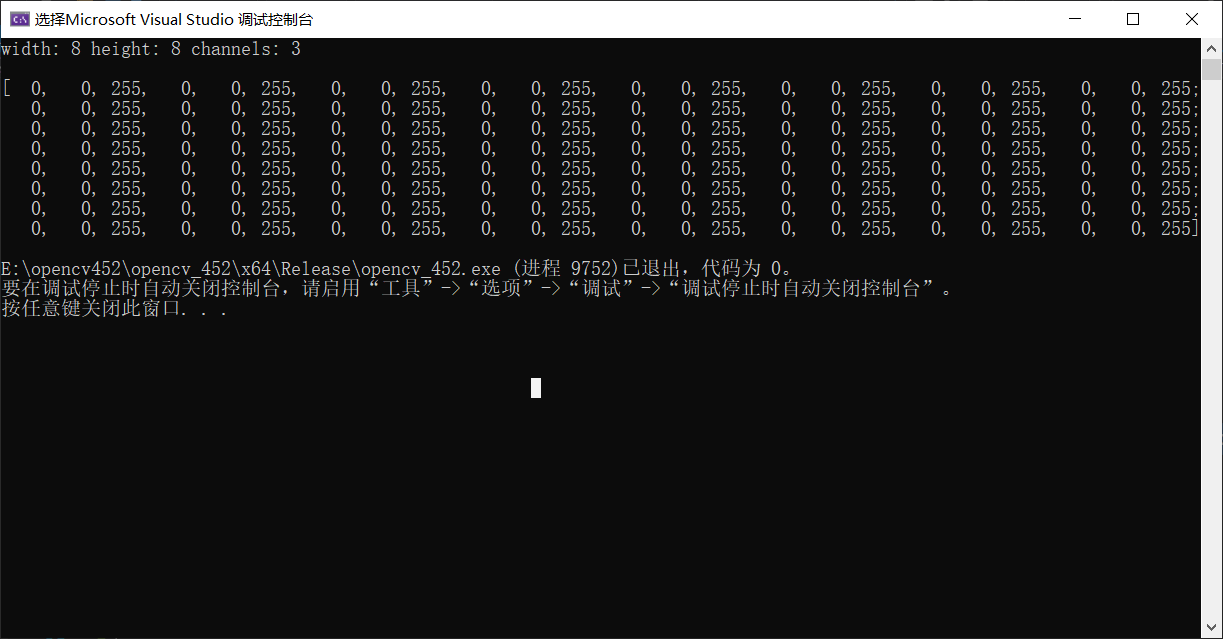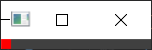• Mat创建图像的理解

千次阅读 2018-06-08 08:54:52
Mat类型： 创建Mat图像处理矩阵的时候，要注意得到的是几个通道的图像。1，若直接Mat image；则创建的是一个任意通道的矩阵，他可以储存任何通道数的转换后的图像，但是！请注意！如果没有用到类似于cvtColor(image,...

Mat类型：

创建Mat图像处理矩阵的时候，要注意得到的是几个通道的图像。

1，若直接Mat image；则创建的是一个任意通道的矩阵，他可以储存任何通道数的转换后的图像，但是！请注意！如果没有用到类似于cvtColor(image,gray,CV_RGB2GRAY)这种转换函数，例如只是用了经过各种操作得到的像素值进行直接赋值，则就会出现错误。因为此时创建的Mat图像的通道数是不确定的，直接赋值显然是错误的。可以直接Mat(image.rows,image.cols,CV_8UC1);则创建的是一个单通道的图像。

CV_8UC1 是指一个8位无符号整型单通道矩阵,

CV_32FC2是指一个32位浮点型双通道矩阵

CV_8UC1           CV_8SC1          CV_16U C1       CV_16SC1
CV_8UC2           CV_8SC2          CV_16UC2        CV_16SC2
CV_8UC3           CV_8SC3          CV_16UC3        CV_16SC3
CV_8UC4           CV_8SC4          CV_16UC4        CV_16SC4
CV_32SC1         CV_32FC1         CV_64FC1
CV_32SC2         CV_32FC2         CV_64FC2
CV_32SC3         CV_32FC3         CV_64FC3

CV_32SC4         CV_32FC4         CV_64FC4

Mat的一个构造函数 C++: Mat::Mat(int rows, int cols, int type, const Scalar& s) ，其中rows和cols是需要创建的矩阵的行数和列数，type是Mat的数据类型，s是Scalar类型的矩阵初值。

对于type，是基本数据类型，首先有枚举 enum { CV_8U=0, CV_8S=1, CV_16U=2, CV_16S=3, CV_32S=4, CV_32F=5, CV_64F=6 }; 分别对应，8位无符号(uchar)、8位有符号(char)、16位无符号(ushort)、16位有符号(short)、32位有符号(int)、32位浮点(float)和64位双精度(double)；其次 CV_8UC1、CV_16FC2、.. CV_64FC4等是多通道的类型，可以用CV_(深度)(类型)(通道数)描述， 例如本例中CV_8UC3，是指8位无符号3通道，其他类推。

对于s的Scalar类型，它的源头实际是一个4行1列的Mat，这里的Scalar(0,0,255)，直接可以理解成M矩阵的每一个元素都是（0，0，255）,当M看成图像，就是一个2x2的红色方块，Scalar有3个值，可以对应RGB色彩,通道顺序为（B,G,R）。那么，CV_8UC2,可以用Scalar(1,2)赋值，CV_64UC4可以用Scalar(0,0.1,0.08,100.1)赋值，其他类推。

展开全文• opencv Mat创建图像

千次阅读 2017-04-11 10:09:13
1、Mat创建、复制 /* * Create Mat */ Mat M(2, 2, CV_8UC3, Scalar(0, 0, 255)); // 构造函数的一种 cout "M=" " " Mat A; M.copyTo(A); M.release(); cout // 释放不影响 Mat B; B =...

1、Mat的创建、复制

/*
* Create Mat
*/
Mat M(2, 2, CV_8UC3, Scalar(0, 0, 255));   // 构造函数的一种
cout << "M=" << endl << " " << M << endl << endl;

Mat A;
M.copyTo(A);

M.release();
cout << A << endl;        // 释放不影响

Mat B;
B = M.clone();

M.release();

cout << "B=" << endl << " " << B << endl<<endl;

Mat src;
src.create(256, 256, CV_8UC(2));

2、Mat的释放

Mat mat1 = Mat::ones(1, 5, CV_32F);
Mat mat2 = mat1;                        // 仅创建一个mat2信息头， mat1，mat2 数据区的地址相同
Mat mat3 = Mat::zeros(1, 4, CV_32F);
mat2.release();          //  因为mat2是对mat1的引用，这里的mat2.release()只会清除mat2的信息头和数据指针
mat1.release();         //  mat1的数据区都会被释放，但是mat信息头数据还会保存（也就是还能继续被赋值）
cout << mat1 << endl;
cout << mat2 << endl;
cout << mat3 << endl << endl;
mat3.copyTo(mat1);// 拷贝会给mat1从新分配数据区域，其原来的数据区还会保留，即mat2的数据是原来mat1的数据，
//mat1 = mat3.clone();  // 最终结果是mat1和mat3的数据相同，但是数据存储空间不同，  mat2存储的是mat1最初的值
mat3.release();        // mat3的释放不会影响mat1
cout << mat1 << endl;
cout << mat2 << endl;

//【14】存取彩色图像的像素
for(int i=0;i<dstDeep1.rows;i++)
{
for(int j=0;j<dstDeep1.cols;j++)
{
dstDeep1.at<Vec3b>(i,j)=255;//蓝色通道
dstDeep1.at<Vec3b>(i,j)=255;//红色通道
dstDeep1.at<Vec3b>(i,j)=255;//绿色通道
}
}

单通道

【14】存取灰度图像的像素
for(int i=0;i<dstDeep1.rows;i++)
{
for(int j=0;j<dstDeep1.cols;j++)
{
dstDeep1.at<uchar>(i,j)=255;//蓝色通道

}
}

展开全文• 使用Mat创建图像（矩阵）的常用形式有： 1.创建一个空图像，大小为0 Mat image1; 2.指定矩阵大小，指定数据类型： Mat image1(100,100,CV_8U); 这里包含三个参数：矩阵行数，矩阵列数，数据类型； 其中数据类型有...

前言

在数字图像处理中，图像的形式实际是一个矩阵，对图像的各种处理也就是对矩阵进行处理。

数字图像处理c++ opencv

一、创建图像（矩阵）：Mat

使用Mat创建图像（矩阵）的常用形式有：
1.创建一个空图像，大小为0

Mat image1;

2.指定矩阵大小，指定数据类型：

Mat image1(100,100,CV_8U);

这里包含三个参数：矩阵行数，矩阵列数，数据类型；
其中数据类型有很多种，常用的应该有：
CV_8U：8位无符号型（0~255），即灰度图像；
CV_8UC3：三通道8位无符号型，这里三通道指B（蓝）G（绿）R（红），与matlab中的RGB正好相反。
这里创建矩阵时未指定矩阵的值，发现默认值的大小为205.

3.指定矩阵大小，指定数据类型，设置初始值：

Mat image1(100,100,CV_8U, 100);

这里包含四个参数：矩阵行数，矩阵列数，数据类型，初始值；
对于灰度图像：可以直接给出初始值，也可以使用Scalar（）；

Mat image1(100,100,CV_8U, 100);
Mat image1(100,100,CV_8U, Scalar(100));

对于三通道图像：使用Scalar（）；

Mat image1(100,100,CV_8UC3, Scalar(100,100,100));

二、获取图像信息

获取图像的宽度（列数），高度（行数），尺寸和通道数：

#include<iostream>
#include<opencv2/opencv.hpp>

using namespace cv;
using namespace std;

int main()
{
if (image1.empty())
{
cout << "读取错误" << endl;
return -1;
}

imshow("image1",image1);  //显示图像；

cout << "图像的行数为： " << image1.rows << endl;  //获取图像的高度，行数；
cout << "图像的列数为： " << image1.cols << endl;  //获取图像的宽度，列数；
cout << "图像的通道数为： " << image1.channels() << endl;  //获取图像的通道数，彩色图=3，灰度图=1；
cout << "图像的尺寸为： " << image1.size << endl;  //获取图像的尺寸，行*列；

waitKey(0);  //暂停，保持图像显示，等待按键结束

return 0;
}

结果：三、感兴趣区域（Rect）

通过Rect()定义一个感兴趣区域：

#include<iostream>
#include<opencv2/opencv.hpp>

using namespace cv;
using namespace std;

int main()
{
if (image1.empty())
{
cout << "读取错误" << endl;
return -1;
}

imshow("image1",image1);  //显示图像；

Mat imageROI(image1, Rect(0,0,10,10));  //定义感兴趣区域

waitKey(0);  //暂停，保持图像显示，等待按键结束

return 0;
}

其中Rect（）有四个参数，Rect（a,b,c,d）:
a：感兴趣区域列(cols)的起点；
b：感兴趣区域行(rows)的起点；
c：感兴趣区域的列数(cols)；
d：感兴趣区域的行数(rows)；

展开全文opencv 计算机视觉 图像处理 c++
• 创建图像对象的四种方法 Mat 对象的创建有多种方式：克隆、复制、赋值、构造函数。 Mat src = imread("d:/test.jpg"); //读取源地址 构造函数 Mat img_constructe(src); 赋值 Mat img_assign = src; ...

MAT

创建图像对象的四种方法

Mat 对象的创建有多种方式：克隆、复制、赋值、构造函数。

 构造函数 Mat img_constructe(src); 赋值 Mat img_assign = src; 复制 Mat img_copy;        src.copyTo(img_copy); 克隆 Mat img_clone = src.clone();

速度从快到慢：构造函数、赋值、复制、克隆。（运行速度代码）

double t1 = (double)getTickCount();

//中间放要测试时间的代码端

t1 = (double)getTickCount() - t1;

//输出运行时间
printf("clone = %lf ms\n", t1*1000. / getTickFrequency());

创建空白图像

创建8*8的矩阵图像  且每个数据单通道

Mat m3 = Mat::zeros(Size(8, 8), CV_8UC1);//创建空图像、8*8矩阵、单通道

std::cout << m3 << std::endl;创建8*8的矩阵图像  且每个数据3通道

Mat m3 = Mat::zeros(Size(8, 8), CV_8UC3);//创建空图像、8*8矩阵、单通道

std::cout << m3 << std::endl;宽度、高度、通道数，且为数据赋值

Mat m3 = Mat::zeros(Size(8, 8), CV_8UC3);//创建空图像、8*8矩阵、3通道

m3 = Scalar(0, 0, 255);

std::cout << "width: " << m3.cols << " height: " << m3.rows << " channels: " << m3.channels() << std::endl;如果输出其图片:BGR（0，0，255）

imshow("图片",m3)

显而易见，创建了一个红色的8*8的图片拷贝与赋值的区别！

拷贝：Mat m4; m3.copyTo(m4);

赋值：Mat m4=m3;

区别：拷贝不会改变原对象，而赋值则m4一变m3也会随之改变！（跟一般int变量不同）

Mat类的函数

data：Mat对象中的一个指针，指向内存中存放矩阵数据的一块内存 (uchar* data)

dims：Mat所代表的矩阵的维度，如 3 * 4 的矩阵为 2 维， 3 * 4 * 5 的为3维

channels：通道，矩阵中的每一个矩阵元素拥有的值的个数，比如说 3 * 4 矩阵中一共 12 个元素，如果每个元素有三个值，那么就说这个矩阵是 3 通道的，即 channels = 3。常见的是一张彩色图片有红、绿、蓝三个通道。

depth：深度，即每一个像素的位数(bits)，在opencv的Mat.depth()中得到的是一个 0 – 6 的数字，分别代表不同的位数：enum { CV_8U=0, CV_8S=1, CV_16U=2, CV_16S=3, CV_32S=4, CV_32F=5, CV_64F=6 }; 可见 0和1都代表8位， 2和3都代表16位，4和5代表32位，6代表64位；

step：是一个数组，定义了矩阵的布局，具体见下面图片分析，另外注意 step1 (step / elemSize1)，M.step[m-1] 总是等于 elemSize，M.step1(m-1)总是等于 channels；

elemSize : 矩阵中每一个元素的数据大小，如果Mat中的数据的数据类型是 CV_8U 那么 elemSize = 1，CV_8UC3 那么 elemSize = 3，CV_16UC2 那么 elemSize = 4；记住另外有个 elemSize1 表示的是矩阵中数据类型的大小，即 elemSize / channels 的大小

展开全文opencv python 计算机视觉
• 一、Mat类的定义：OpenCV3 参考文档：cv::Mat Class Mat类的对象用于表示一个多维度的单通道或者多通道稠密数组，它可以用来存储以下东西： real or complex-valued vectors or matrices(实数或复数的向量或者...opencv3 图像处理
• 实验说明： （引用） ...　本文主要讲一些opencv 2.0版本后出现的Mat矩形类，主要是参考opencv自带doc文件夹下的tutiol教材。通过这次实验觉得用Mat的话下面几点需要特别注意（在代码中可以体现出来）： 1. 利
• Mat¶在2001年刚刚出现的时候，OpenCV基于 C 语言接口而建。为了在内存(memory)中存放图像，当时采用名为 IplImage 的C语言结构体，时至今日这仍出现在大多数的旧版教程和教学材料。但这种方法必须接受C语言所有的...
• 我们在实际应用中对图像进行的操作，往往并...Mat类提供了一个at的方法用于取得图像上的点，它是一个模板函数，可以取到任何类型的图像上的点。下面我们通过一个图像处理中的实际来说明它的用法。 在实际应用中，我计算机视觉 OpenCV 图像处理
• 在学习笔记(1)中已经提到opencv2.x及3.x中用Mat代替了CvMat和IplImage，也就是说Mat既可以代替CvMat类型矩阵数据，也可以代替IplImage类型的图像数据，也就是说Mat统一了前两中数据结构。因此在OpenCv2中对矩阵数据...opencv mat 显示
• opencv Mat选取图像局部区域

千次阅读 2018-11-28 16:45:02
Mat 类提供了多种方便的方法来选择图像的局部区域。使用这些方法时需要注意,这些方法并不进行内存的复制操作。如果将局部区域赋值给新的 Mat 对象,新对象与原始对象共用相同的数据区域,不新申请内存,因此这些方法的...Mat::row
• OpenCV Mat矩阵(图像Mat)初始化及访问方法

万次阅读 多人点赞 2019-06-20 17:39:50
一、Mat初始化 1.使用Mat构造函数 //方法一： Mat M( 2, 2, CV_8UC3, Scalar(0,255,0) );//其实是2*6的矩阵，因为每个元素有3个通道。 Mat M1( 2, 2, CV_8UC1，Scalar(0) );//单通道 //方法二： int sz = {2, 2, ...
• 1、一个Mat对象（图片）包含头部和数据部分两部分信息。 2、当使用赋值方式时，并不会重新开辟一块空间来存储这张图片的信息。Mat还有一个克隆/拷贝方法，当调用这两个方法时，才会将另外开辟一块...如何创建空白图像..opencv
• 1. Mat数据类型 Mat定义 是一个类，由两个数据部分构成，矩阵头（包含矩阵尺寸，存储方法，存储地址等等）和一个指向存储所有像素值的矩阵的指针 成员变量 int cv::Mat::cols; //返回矩阵的列数 int cv::Mat::...C
• opencv创建Mat对象

千次阅读 2019-03-31 17:36:04
Mat类是一个非常优秀的图像类，它同时也是一个通用的矩阵类，可以用来创建和操作多维矩阵。Mat类里面定义了许多构造函数，可以很方便地根据要求创建Mat对象。 常使用的构造函数有： 1：Mat::Mat()无参数构造函数；...
• 1. Mat数据类型 Mat定义 是一个类，由两个数据部分构成，矩阵头（包含矩阵尺寸，存储方法，存储地址等等）和一个指向存储所有像素值的矩阵的指针 成员变量 int cv::Mat::cols; //返回矩阵的列数 int cv::Mat::rows ...
• Mat类是一个储存矩阵数据的容器，包括灰度、向量、矩阵、彩色图像等数据。Mat类分为矩阵头和指向存储数据的矩阵指针两部分。 矩阵头： 矩阵尺寸： 存储方法: 地址： 引用次数: 而图像传递和复制过程中，主要...opencv 人工智能 自动驾驶
• OpenCV 创建图像 # -*- coding: utf-8 -*- # @Time : 2021/7/20 # @Author : ZYh """ Introduction: 创建图像: ·OpenCV中，黑白图像实际是一个二维数组，彩色图像是一个三维数组。 数组中每个元素就是图像对应...opencv numpy 计算机视觉
• opencv（mat与qimage 的互转）及图像处理一、opencv库文件二、使用方式（Qt）三、QImage 转 Mat (不太全，多多指教)四、Mat转 QImage总结 一、opencv库文件 编译好的opencv 库。 二、使用方式（Qt） 把上面下载的...opencv 图像处理 计算机视觉
• I would have thought this is trivial, but I'm having some trouble with it.I want to read a video file into memory and store it in an ... I want the array to be of pointers to Mat objects.This is th...
• 1、使用Mat()构造函数定义一个二维多通道图像Mat M(2, 2, CV_8UC3, Scalar(0, 0, 255));cout << "M = " << endl << " " << M << endl;/**2行2列* CV_8UC3 :使用8为unsigned char型，...
• cv::Mat初识和它的六种创建方法

万次阅读 多人点赞 2019-06-04 14:52:49
cv::Mat 初识   Opencv种的Mat类，使得Opencv的编程更加的简单，程序员不用过多的去关注内存管理，并且发现Opencv的编程有点像不需要太多编程技术的Matlab一样，甚至有些函数名字都是一样的。所以对Mat的了解是很...cv::Mat opencv c/c++
• 创建mat对象的几种方法

千次阅读 2018-05-06 15:17:34
//创建三个通道的图像，并为其定义尺寸、及通道的初始值方法二：通过构造函数进行初始化int sz = { 2,2,2 }; Mat L(3, sz, CV_8UC1, Scalar::all(0));此方法可以定义超过二位的矩阵；第一个参...
• 对于s的Scalar类型，它的源头实际是一个4行1列的Mat，这里的Scalar(0,0,255)，直接可以理解成M矩阵的每一个元素都是（0，0，255）,当M看成图像，就是一个2x2的红色方块，Scalar有3个值，可以对应RGB色彩,通道顺序为...mat 释放
• 图像和矩阵： 现实中我们看到的图像是这样...C++操作矩阵的库有很多,机器视觉方向最火的当属OpenCV,其图像容器Mat提供了非常方便的矩阵操作来处理数字化图像Mat不但是一个非常有用的图像容器类,同时也是一个通用...opencv c++
• 个人记录，不喜勿喷。... cv::Mat img = cv::Mat::zeros(rows, cols, CV_8UC1);/* zeros可有可无 */ for (int row = 0; row < rows; row++) { uchar* uc = img.data + row * img.step; for (int colOpenCV 创建图像
• Mat类可以被看做是opencv中C++版本的矩阵类，替代原来C版本的矩阵结构体CvMat和图像结构体IplImage； Mat最大的优势跟STL的兼容性很好，有很多类似于STL的操作。但是Mat远远强于后者； Mat是一种高效的数据类型，...opencv c++ 人工智能
• opencv图像读取和cv::Mat

千次阅读 2017-05-26 15:25:40opencvopencv Mat 图像连接
• 创建Mat 对象

千次阅读 2013-03-01 13:31:15
为此，你可以通过 Mat 的运算符 Mat 不但是一个很赞的图像容器类，它同时也是一个通用的矩阵类，所以可以用来创建和操作多维矩阵。创建一个Mat对象有多种方法：矩阵。创建一个Mat对象有多种方法：Mat() 构造函数对于...OpenCV...